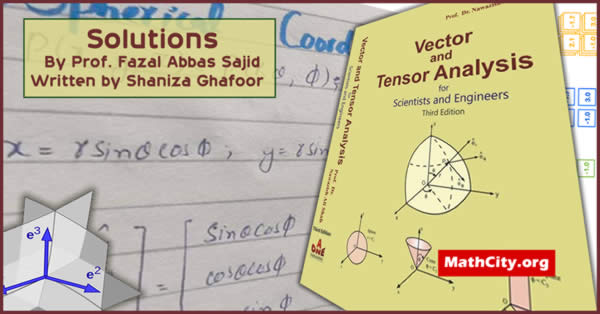# Vector & Tensor Analysis by Dr Nawazish Ali (Solutions)We are very thankful to Prof. Fazal Abbas Sajid for sharing these solutions. Vector & Tensor Analysis for Scientists and Engineers, by Prof. Dr. Nawazish Ali Shah is a famous book taught in different universities of the Pakistan. On this page, we have added the solutions of the exercises of the book. Solutions of Chapter 5 is written by Ms. Javeria Abbas Ahmand and Chapter 6 is written by Ms. Shaniza Ghafoor.

 Name Vector & Tensor Analysis (Solutions) Prof. Fazal Abbas Sajid PDF (see Software section for PDF Reader) different chapters have different sizes
• Curvilinear coordinates
• Transformation of coordinates
• Coordinate surfaces and coordinate curves
• Unit vectors in curvilinear coordinate system
• Rectangular Cartesian coordinates
• Expressions for arc length, area and volume elements in rectangular Cartesian coordinates
• Expression for Jacobian in rectangular Cartesian coordinates
• Expression for gradient, divergence, curl and Laplacian in rectangular Cartesian coordinates
• Cylindrical polar coordinate
• Cylindrical coordinates in terms of Cartesian coordinates
• Unit vectors in cylindrical coordinate system
• Orthogonally of cylindrical coordinate system
• Relationship among unit vector in cylindrical system
• Chapter 5: Line, Surface, Volume Integral and Related Integral Theorems | Download PDF ~ 8.94MB |

• msc/notes/vector-and-tensor-analysis-by-dr-nawazish